Conge 精进

# RL 阅读材料笔记 Littman (1996) Chapters 1-2

2015-09-01

Littman, 1996, chapter 1,2

# Chapter 1: Introduction

## 1.1 Core concepts of Sequential decision making

Purpose: producing policies that maximize a measure of long-term reward to an agent following it in a specific environment.

### Elements of Sequential decision making

• Agent: the system responsible for interacting with the world and making decisions.
• Environment: change from state to state (s) in response to the actions (a) of the agent according to a fixed set of rules (T). Environment can be stationary(<-main focus of the paper) or nonstationary (<-hard to model). Environment can have multiple agents.
• Reward: the purpose of agents’ action is to maximize rewards. Reward is a defining property of models.
• Policies: Agent’s prescription for actions. plan-> conditional plan -> universal plan or “stationary policy” -> stochastic policy. Stationary policy is the most important one in this paper.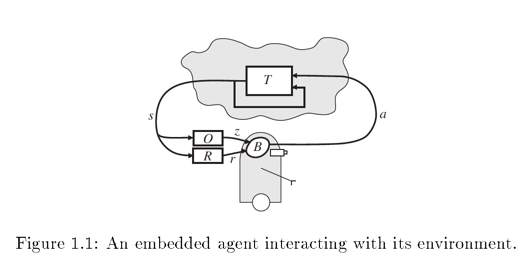In the figure above, s is state, a is action, T is transition function which changes s given a. R is the reward function and r is the reward of the state. O is perception function (usually it is an identity function) and z is the agent’s perception of the environment.

### Problem scenarios：

• Planning: the complete model of the environment is known and a planner takes the model and generate policy.
• Reinforcement Learning (RL): the learner only knows the agent’s perception of the world and action it can take. Environment is unknown. It’s up to the agent to generate a policy and follow it.
• Model based RL: building a model with the limited information known to the agent, and then use a planning algorithm to generate a policy.
• Simulated RL: in this case, the model is known but the agent acts like it is not known and use RL algorithm to generate policy. It is used when the environment is too complicated.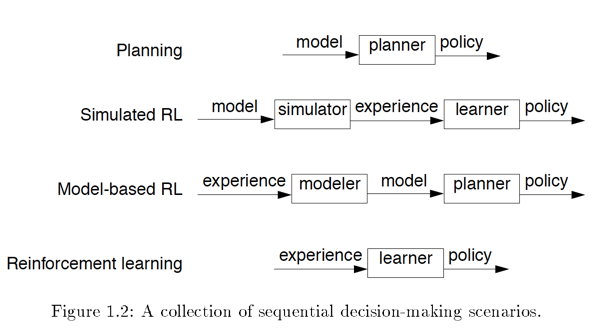## 1.2 Formal Models

To simplify the problem, the properties of the environment model must follow the following conditions:

finite state space; finite actions; sequential environment; accessible and inaccessible environment; Markovian environment (prediction can be made by environment’s state); fixed environment; stochastic or deterministic transitions; synchronous environment ( environment won’t change until the agent takes an action); with one or two agent.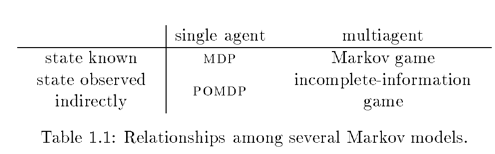## 1.3 evaluation criteria

Evaluating Policies: an objective function takes all the <s, a> sequences and their probabilities to generate value. The optimal sequence maximize the objective function. 1) for each <s, a> sequences, objective function replace each transition with a “goodness” value; 2) the values are truncated according to the finite horizon; 3) transition values were summarized for each sequence; 4) this sequence value are summarized to get a policy value; 5) in multiagent environment, other agent can change transition value.

Evaluating Planning algorithms: 1) All criteria above; 2) whether an optimal policy can be reached; 3) algorithm efficiency.

** RL algorithms**: RL algorithms tries to achieve what planning can do without complete knowledge of the whole environment. If the algorithm can reach optimal policy (converge), then it’s good.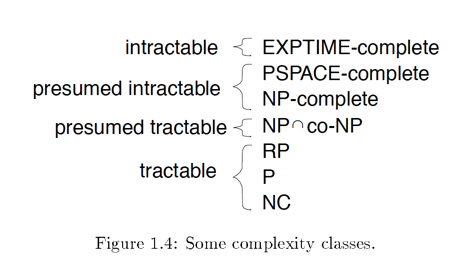# Chapter 2: Markov Decision Process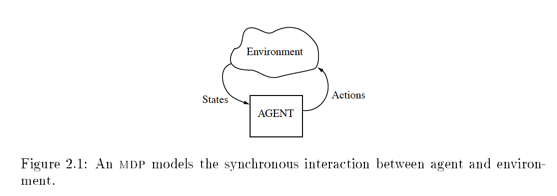Core concept of MDP below is extracted from my notes of the course lecture.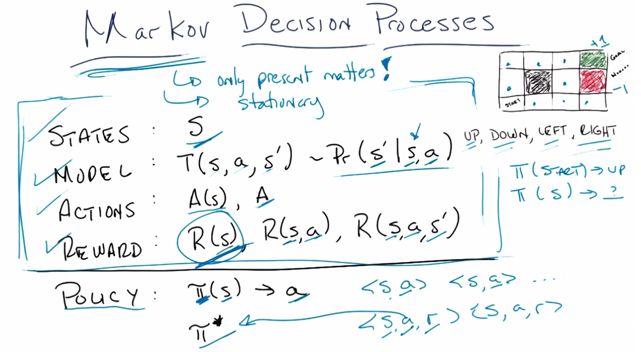States: set of tokens which describe every state that one could be in,

Model/Transition Function: probability that you’ll end up transition to s’ given you are in state s and taking action a. This is ‘physics’ or rules of the world(not changeable) .

Action: A(s), things you can do in a given state, a function of state, rule you can play; or A, a set actions not depends regardless of state.

Reward: scalar value that you get for being in a state. Usefulness of entering a state/and taking an action/and ending up into s’

S, T, A and R define the problem, policy is the solution,

Policy: the solution, a function that takes up a state and tells an action that you’ll take <s,a> while a is the correct action you want to take to maximize the reward

*Special policy:  π*, optimized, maximize long term expected reward. (note, from <s,a,r>, you know s, a and then know the r, based on rewards, find π*) r–>z s–>x a–>y, π–>f

## Algorithms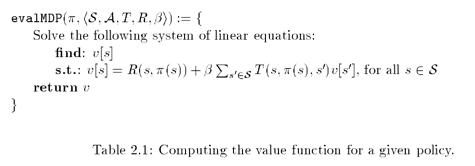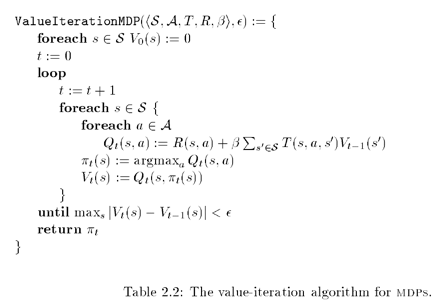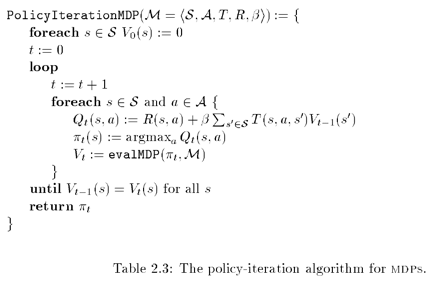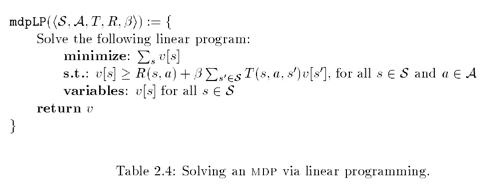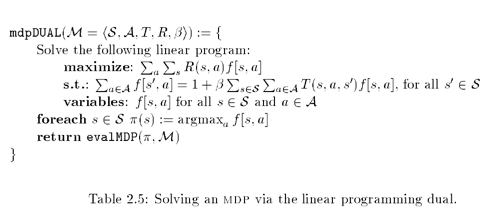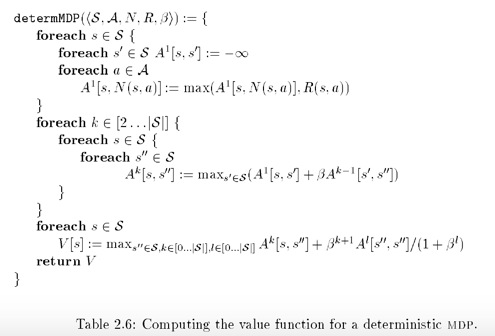``````2015-08-26 第一章完成

``````